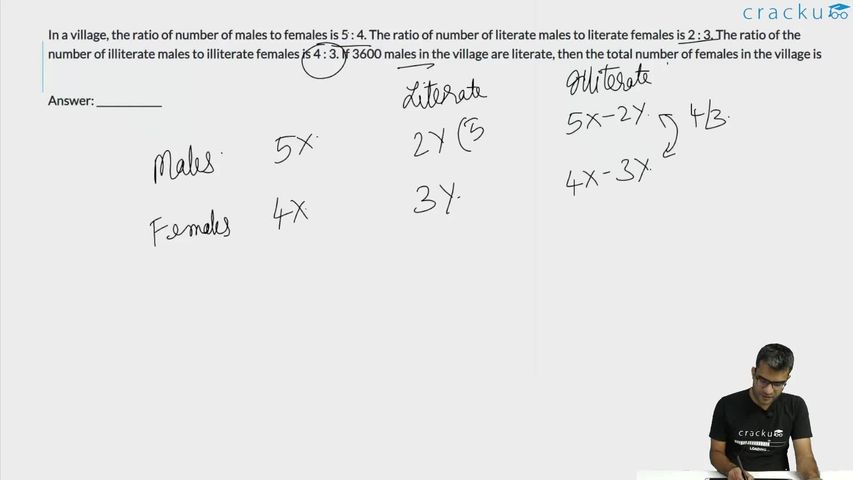Question 45

# In a village, the ratio of number of males to females is 5 : 4. The ratio of number of literate males to literate females is 2 : 3. The ratio of the number of illiterate males to illiterate females is 4 : 3. If 3600 males in the village are literate, then the total number of females in the village is

Solution

In the question, it is given that the ratio of number of literate males to literate females is 2 : 3.

Given, the number of literate males = 3600

The number of literate females = $$\frac{3600}{2}\times3$$ = 5400

Males : Females = 5 : 4

Let the number of males be 5y and the number of females be 4y

Illiterate males = 5y - 3600

Illiterate females = 4y - 5400

It is given,

$$\ \frac{\ 5y-3600}{4y-5400}=\frac{4}{3}$$

15y - 10800 = 16y - 21600

y = 10800

Number of females = 4y = 4*10800 = 43200

### View Video Solution• All Quant CAT Formulas and shortcuts PDF
• 30+ CAT previous papers with solutions PDF